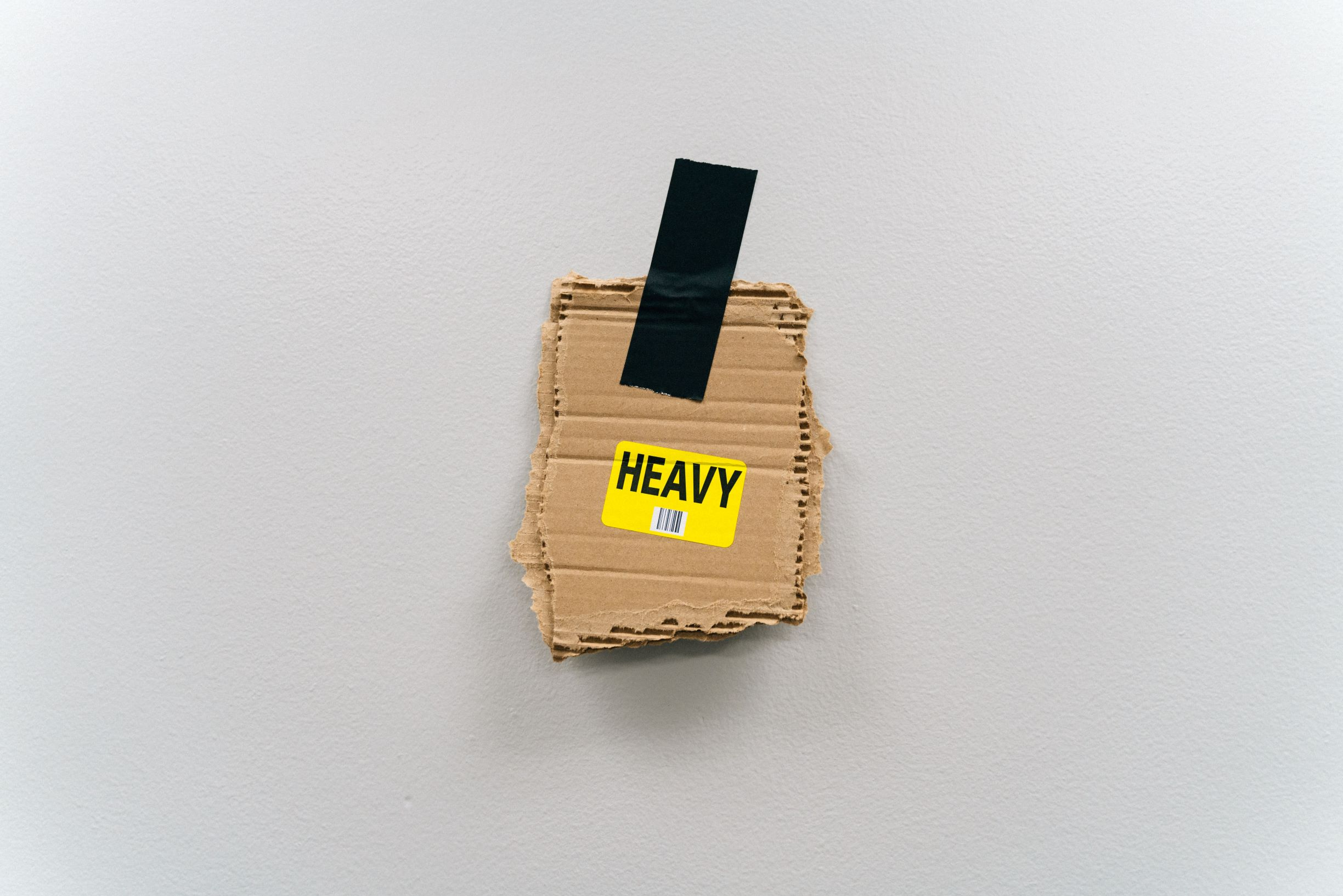# June 2019 DIM Weight USPS Rate Change

July 11, 2019On June 23, 2019 the USPS enacted a second rate change.  They will start using dimensional weight to calculate the package pricing for Priority Mail®, Priority Mail Express® and Parcel Select. They are also changing the way the calculate dimensional weight. Continue reading for a more detailed breakdown.

Dimensional (DIM) Weight
All major carriers (UPS, FedEx and now USPS) use dimensional weight to determining the shipping costs of larger packages with lower weights, which means light packages that take up valuable space in delivery trucks and airplanes. If the calculated dimensional weight is higher than the actual weight,you will be required the higher amount.

Dimensional Weight Calculation

There are several ways to calculate dimensional weight: so you can calculate it by hand, by using Neopost’s Online Calculator or Neoship online shipping software.

Some prefer to double check the work of computers so you calculate dimensional weight on your own using the following rules.

1. Multiply the length, width, and height of your parcel. Determine if it is over 1 cubic foot or 1,728 cubic inches.
2. If your package is less than 1,728 cubic inches, you don’t need to enter in the dimensional weight. Your package will be priced off of the actual weight.
3. If your package is greater than 1,728 cubic inches, you will divide the cubic inches by 166 to calculate the weight of your package.

#### How Do You Measure Dimensional Weight?

Below is an example Neopost provides on how to calculate dimensional weight:

Example 1: You are shipping sneakers that weight 7lbs. in a box that measures 12″ x 12″ x 8″. Multiply the length x width x height of the package: 12″ x 12″ x 8″= 1,056 cubic inches. You will calculate the price using the actual weight of 7 lbs. because your package is under 1 cubic foot or 1,728 cubic inches.

Example 2: You are shipping a book that weighs 5 lbs. in a box that measures 12″ x 12″ x 14″. You multiply the length x width x height of the package. 12″ x 12″ x 14″ = 2,016 cubic inches. The dimensions are over 1 cubic foot or 1,728 cubic inches, so you will then divide the dimensions by the USPS divisor which is 166. 2,016 cubic inches / 166 = 12.14 lbs. rounded up to 13 lbs. This is the weight you will enter into the machine.

The fees can really add up if you are shipping large, lightweight packages! To reduce being charged for dimensional weight, you can use:

• The smallest packaging possible
• Flat Rate boxes and envelopes
• Padded envelopes for flexible items like shipping clothing